Physics of Hearing

# Speed of Sound, Frequency, and Wavelength

OpenStaxCollege

### Learning Objectives

• Define pitch.
• Describe the relationship between the speed of sound, its frequency, and its wavelength.
• Describe the effects on the speed of sound as it travels through various media.
• Describe the effects of temperature on the speed of sound.
When a firework explodes, the light energy is perceived before the sound energy. Sound travels more slowly than light does. (credit: Dominic Alves, Flickr)Sound, like all waves, travels at a certain speed and has the properties of frequency and wavelength. You can observe direct evidence of the speed of sound while watching a fireworks display. The flash of an explosion is seen well before its sound is heard, implying both that sound travels at a finite speed and that it is much slower than light. You can also directly sense the frequency of a sound. Perception of frequency is called pitch. The wavelength of sound is not directly sensed, but indirect evidence is found in the correlation of the size of musical instruments with their pitch. Small instruments, such as a piccolo, typically make high-pitch sounds, while large instruments, such as a tuba, typically make low-pitch sounds. High pitch means small wavelength, and the size of a musical instrument is directly related to the wavelengths of sound it produces. So a small instrument creates short-wavelength sounds. Similar arguments hold that a large instrument creates long-wavelength sounds.

The relationship of the speed of sound, its frequency, and wavelength is the same as for all waves: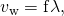where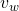is the speed of sound,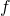is its frequency, and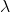is its wavelength. The wavelength of a sound is the distance between adjacent identical parts of a wave—for example, between adjacent compressions as illustrated in [link]. The frequency is the same as that of the source and is the number of waves that pass a point per unit time.

A sound wave emanates from a source vibrating at a frequency, propagates at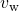, and has a wavelength.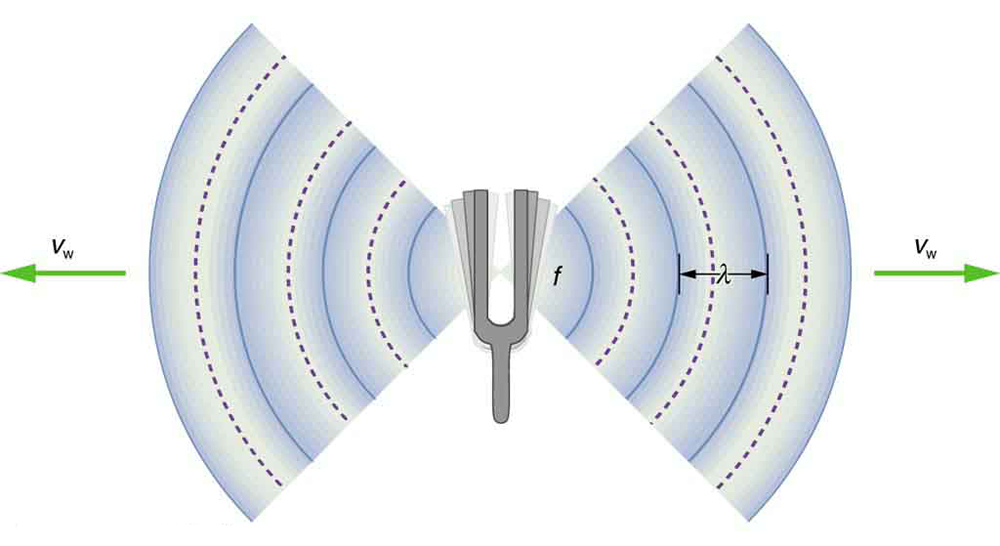[link] makes it apparent that the speed of sound varies greatly in different media. The speed of sound in a medium is determined by a combination of the medium’s rigidity (or compressibility in gases) and its density. The more rigid (or less compressible) the medium, the faster the speed of sound. This observation is analogous to the fact that the frequency of a simple harmonic motion is directly proportional to the stiffness of the oscillating object. The greater the density of a medium, the slower the speed of sound. This observation is analogous to the fact that the frequency of a simple harmonic motion is inversely proportional to the mass of the oscillating object. The speed of sound in air is low, because air is compressible. Because liquids and solids are relatively rigid and very difficult to compress, the speed of sound in such media is generally greater than in gases.

Speed of Sound in Various Media
Medium vw(m/s)
Gases at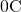Air 331
Carbon dioxide 259
Oxygen 316
Helium 965
Hydrogen 1290
Liquids at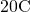Ethanol 1160
Mercury 1450
Water, fresh 1480
Sea water 1540
Human tissue 1540
Solids (longitudinal or bulk)
Vulcanized rubber 54
Polyethylene 920
Marble 3810
Glass, Pyrex 5640
Lead 1960
Aluminum 5120
Steel 5960

Earthquakes, essentially sound waves in Earth’s crust, are an interesting example of how the speed of sound depends on the rigidity of the medium. Earthquakes have both longitudinal and transverse components, and these travel at different speeds. The bulk modulus of granite is greater than its shear modulus. For that reason, the speed of longitudinal or pressure waves (P-waves) in earthquakes in granite is significantly higher than the speed of transverse or shear waves (S-waves). Both components of earthquakes travel slower in less rigid material, such as sediments. P-waves have speeds of 4 to 7 km/s, and S-waves correspondingly range in speed from 2 to 5 km/s, both being faster in more rigid material. The P-wave gets progressively farther ahead of the S-wave as they travel through Earth’s crust. The time between the P- and S-waves is routinely used to determine the distance to their source, the epicenter of the earthquake.

The speed of sound is affected by temperature in a given medium. For air at sea level, the speed of sound is given by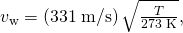where the temperature (denoted as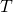) is in units of kelvin. The speed of sound in gases is related to the average speed of particles in the gas,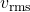, and that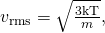where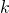is the Boltzmann constant (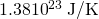) and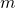is the mass of each (identical) particle in the gas. So, it is reasonable that the speed of sound in air and other gases should depend on the square root of temperature. While not negligible, this is not a strong dependence. At, the speed of sound is 331 m/s, whereas at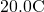it is 343 m/s, less than a 4% increase. [link] shows a use of the speed of sound by a bat to sense distances. Echoes are also used in medical imaging.

A bat uses sound echoes to find its way about and to catch prey. The time for the echo to return is directly proportional to the distance.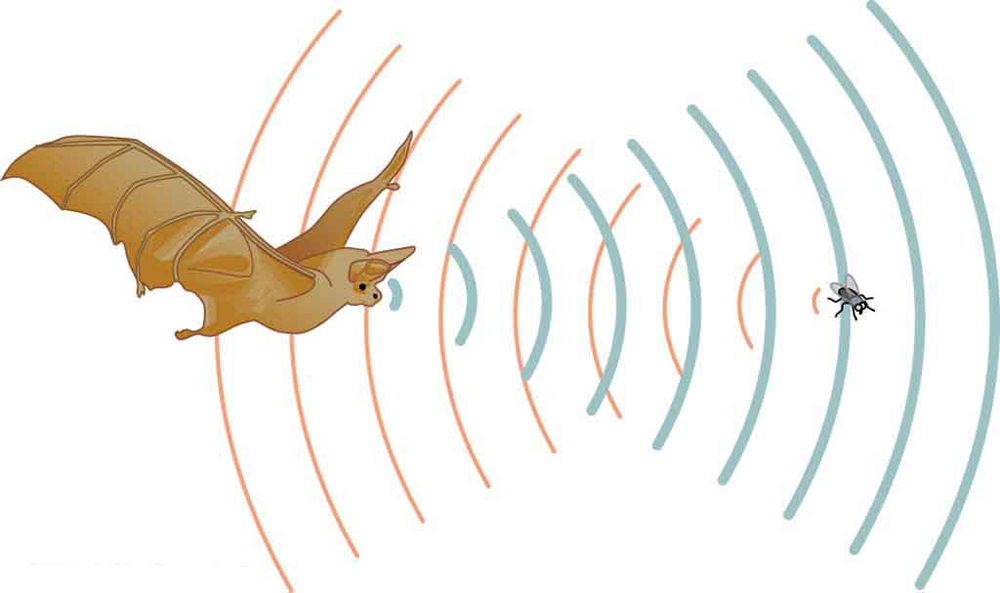One of the more important properties of sound is that its speed is nearly independent of frequency. This independence is certainly true in open air for sounds in the audible range of 20 to 20,000 Hz. If this independence were not true, you would certainly notice it for music played by a marching band in a football stadium, for example. Suppose that high-frequency sounds traveled faster—then the farther you were from the band, the more the sound from the low-pitch instruments would lag that from the high-pitch ones. But the music from all instruments arrives in cadence independent of distance, and so all frequencies must travel at nearly the same speed. Recall that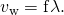In a given medium under fixed conditions,is constant, so that there is a relationship betweenand; the higher the frequency, the smaller the wavelength. See [link] and consider the following example.

Because they travel at the same speed in a given medium, low-frequency sounds must have a greater wavelength than high-frequency sounds. Here, the lower-frequency sounds are emitted by the large speaker, called a woofer, while the higher-frequency sounds are emitted by the small speaker, called a tweeter.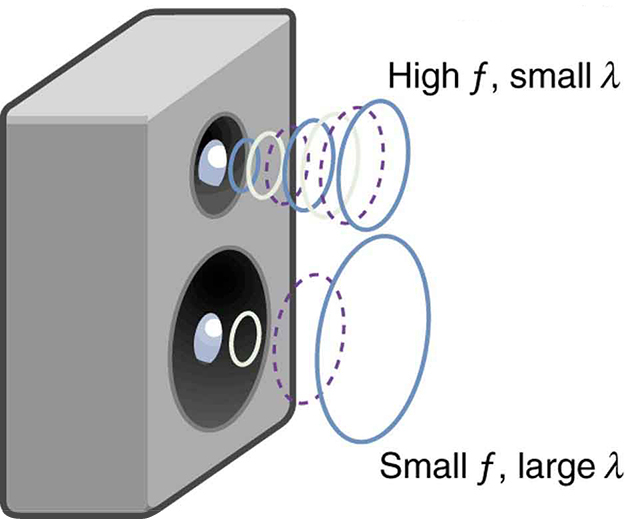Calculating Wavelengths: What Are the Wavelengths of Audible Sounds?

Calculate the wavelengths of sounds at the extremes of the audible range, 20 and 20,000 Hz, in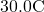air. (Assume that the frequency values are accurate to two significant figures.)

Strategy

To find wavelength from frequency, we can use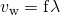.

Solution

1. Identify knowns. The value for, is given by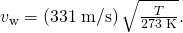2. Convert the temperature into kelvin and then enter the temperature into the equation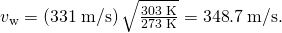3. Solve the relationship between speed and wavelength for: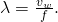4. Enter the speed and the minimum frequency to give the maximum wavelength: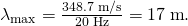5. Enter the speed and the maximum frequency to give the minimum wavelength: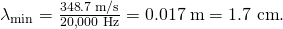Discussion

Because the product ofmultiplied byequals a constant, the smalleris, the largermust be, and vice versa.

The speed of sound can change when sound travels from one medium to another. However, the frequency usually remains the same because it is like a driven oscillation and has the frequency of the original source. Ifchanges andremains the same, then the wavelengthmust change. That is, because,

the higher the speed of a sound, the greater its wavelength for a given frequency.

Making Connections: Take-Home Investigation—Voice as a Sound Wave

Suspend a sheet of paper so that the top edge of the paper is fixed and the bottom edge is free to move. You could tape the top edge of the paper to the edge of a table. Gently blow near the edge of the bottom of the sheet and note how the sheet moves. Speak softly and then louder such that the sounds hit the edge of the bottom of the paper, and note how the sheet moves. Explain the effects.

Check Your Understanding

Imagine you observe two fireworks explode. You hear the explosion of one as soon as you see it. However, you see the other firework for several milliseconds before you hear the explosion. Explain why this is so.

Sound and light both travel at definite speeds. The speed of sound is slower than the speed of light. The first firework is probably very close by, so the speed difference is not noticeable. The second firework is farther away, so the light arrives at your eyes noticeably sooner than the sound wave arrives at your ears.

Check Your Understanding

You observe two musical instruments that you cannot identify. One plays high-pitch sounds and the other plays low-pitch sounds. How could you determine which is which without hearing either of them play?

Compare their sizes. High-pitch instruments are generally smaller than low-pitch instruments because they generate a smaller wavelength.

# Section Summary

The relationship of the speed of sound, its frequency, and its wavelengthis given by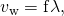which is the same relationship given for all waves.

In air, the speed of sound is related to air temperaturebyis the same for all frequencies and wavelengths.

# Conceptual Questions

How do sound vibrations of atoms differ from thermal motion?

When sound passes from one medium to another where its propagation speed is different, does its frequency or wavelength change? Explain your answer briefly.

# Problems & Exercises

When poked by a spear, an operatic soprano lets out a 1200-Hz shriek. What is its wavelength if the speed of sound is 345 m/s?

0.288 m

What frequency sound has a 0.10-m wavelength when the speed of sound is 340 m/s?

Calculate the speed of sound on a day when a 1500 Hz frequency has a wavelength of 0.221 m.

332 m/s

(a) What is the speed of sound in a medium where a 100-kHz frequency produces a 5.96-cm wavelength? (b) Which substance in [link] is this likely to be?

Show that the speed of sound inair is 343 m/s, as claimed in the text.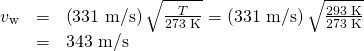Air temperature in the Sahara Desert can reach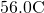(about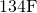). What is the speed of sound in air at that temperature?

Dolphins make sounds in air and water. What is the ratio of the wavelength of a sound in air to its wavelength in seawater? Assume air temperature is.

0.223

A sonar echo returns to a submarine 1.20 s after being emitted. What is the distance to the object creating the echo? (Assume that the submarine is in the ocean, not in fresh water.)

(a) If a submarine’s sonar can measure echo times with a precision of 0.0100 s, what is the smallest difference in distances it can detect? (Assume that the submarine is in the ocean, not in fresh water.)

(b) Discuss the limits this time resolution imposes on the ability of the sonar system to detect the size and shape of the object creating the echo.

(a) 7.70 m

(b) This means that sonar is good for spotting and locating large objects, but it isn’t able to resolve smaller objects, or detect the detailed shapes of objects. Objects like ships or large pieces of airplanes can be found by sonar, while smaller pieces must be found by other means.

A physicist at a fireworks display times the lag between seeing an explosion and hearing its sound, and finds it to be 0.400 s. (a) How far away is the explosion if air temperature is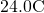and if you neglect the time taken for light to reach the physicist? (b) Calculate the distance to the explosion taking the speed of light into account. Note that this distance is negligibly greater.

Suppose a bat uses sound echoes to locate its insect prey, 3.00 m away. (See [link].) (a) Calculate the echo times for temperatures of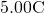and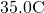. (b) What percent uncertainty does this cause for the bat in locating the insect? (c) Discuss the significance of this uncertainty and whether it could cause difficulties for the bat. (In practice, the bat continues to use sound as it closes in, eliminating most of any difficulties imposed by this and other effects, such as motion of the prey.)

(a) 18.0 ms, 17.1 ms

(b) 5.00%

(c) This uncertainty could definitely cause difficulties for the bat, if it didn’t continue to use sound as it closed in on its prey. A 5% uncertainty could be the difference between catching the prey around the neck or around the chest, which means that it could miss grabbing its prey.

## Glossary

pitch
the perception of the frequency of a sound

## LicenseSpeed of Sound, Frequency, and Wavelength by OpenStaxCollege is licensed under a Creative Commons Attribution 4.0 International License, except where otherwise noted.